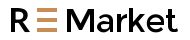Free Shipping on orders over US\$39.99

# Instrumentation Amplifier Offset Voltage and Noise Technical Analysis

Since there are offset voltages in the two-stage amplifiers inside the instrumentation amplifier, such as the offset voltage of the first-stage amplifier where AMP1 and AMP2 are located in Figure 3.1, if it is converted to the output end, it needs to be multiplied by the circuit gain. If the offset voltage of the second stage amplifier where AMP3 is located, if converted to the input end, it needs to be divided by the circuit gain.

Author: Zheng Huimin

Instrumentation Amplifier Offset Voltage Analysis

Since there are offset voltages in the two-stage amplifiers inside the instrumentation amplifier, such as the offset voltage of the first-stage amplifier where AMP1 and AMP2 are located in Figure 3.1, if it is converted to the output end, it needs to be multiplied by the circuit gain. If the offset voltage of the second stage amplifier where AMP3 is located, if converted to the input end, it needs to be divided by the circuit gain.Figure 3.1 Instrumentation amplifier structure

The above-mentioned offset voltage of the first-stage amplifier is called an input offset voltage (Input Offset Voltage, VOSI), and the offset voltage of the second-stage amplifier is called an output offset voltage (Output Offset Voltage, VOSO). The total output offset voltage Total Vos_RTO is Equation 3-8.where G is the gain. This is also why the linearity error of an instrumentation amplifier changes after switching gains.

As shown in Figure 3.10, taking AD8421ARZ as an example, in a 25°C environment, using ±15V power supply, the maximum input offset voltage is 60μV, and the maximum output offset voltage is 350μV. In a circuit with a gain of 1, the AD8421ARZ outputs a maximum total offset voltage of 410uV. In a circuit with a gain of 100, the total output offset voltage is a maximum of 6.35mV.As shown in Figure 3.11(a), the AD8421 is designed with LTspice at a gain of 1, and the power supply is ±15V. As shown in Figure 3.11(b), the transient analysis result of the output total offset voltage is 412.292μV, which is equivalent to the theoretical calculated value.Figure 3.11 Total output offset voltage transient analysis results for AD8421 gain 1 circuit

As shown in Figure 3.12(a), use LTspice to design a circuit with AD8421 supplying ±15V and a gain of 100 times. The output total offset voltage transient analysis result, as shown in Figure 3.12(b), is 6.353mV, which is equivalent to the theoretical calculation value.3.12 Transient analysis results of total output offset voltage of AD8421 gain of 100 times circuit

Instrumentation Amplifier Noise Analysis

There are two sources of voltage noise in instrumentation amplifiers. One is the noise source ENI connected in series at the input, like a general-purpose amplifier. Converted to the output by the circuit gain. The second is the noise voltage source ENO connected in series at the output end of the instrumentation amplifier, which is converted to the input end by dividing by the gain of the circuit. As shown in Figure 3.13, in a 25°C environment, when powered by a ±15V power supply, the maximum input voltage noise density eni of AD8421 at 1KHz is 3.2 nV/√Hz, and the maximum output voltage noise density eno is 60nV/√Hz.There are also two sources of current noise at the input of the instrumentation amplifier, iN+ and iNC. Although the two current noises are approximately equal. As shown in Figure 3.13, in a 25°C environment, when powered by a ±15V power supply, the AD8421 current noise spectral density in at 1KHz is a maximum of 0.2 pA/√Hz. But iN+ and iNC are not related and must be summed in a root mean square manner. iN+ flows through half of the signal source resistance RS, and iNC flows through the other half of the signal source resistance RS. The two resulting noise voltages are each half the magnitude of INRS. These two noise sources are converted to the output by the circuit gain.

The gain resistor of the instrumentation amplifier also creates a noise source, the output of which is reduced by the gain of the circuit.

From this, the RMS value of the total output noise of the instrumentation amplifier is Equation 3-9.Among them, BW is 1.57 times the signal bandwidth.

As shown in Figure 3.14(a), the AD8421 is used to configure a circuit with a gain of 1 (Rg canceled), the internal resistance of the signal source is set to 0, and within the frequency of 0.1~10KHz, the maximum output noise voltage RMS is about:Figure 3.14 The circuit noise analysis results with AD8421 gain of 1

The noise analysis result is shown in Figure 3.14(b), the total output noise RMS value of AD8421 is 5.59μV and less than 7.52μV.

As shown in Figure 3.15(a), the circuit configuration is 100 times gain (Rg is 100Ω), the internal resistance of the signal source is set to 0, and within the frequency of 0.1~10KHz, the maximum output noise voltage RMS is approximately:The noise analysis result is shown in Figure 3.15(b). The total output noise of AD8421 is 34.315μV, which is less than 43.76μV.Figure 3.15 Noise analysis of AD8421 circuit with a gain of 100 times

To sum up, when analyzing the offset voltage and noise parameters of the instrumentation amplifier, the offset voltage, noise and gain of the circuit configuration of the internal two-stage amplifier are converted to the input or output to evaluate the total offset voltage and total noise.

(Source: Amplifier parameter analysis and LTspice simulation, author: Zheng Huimin)